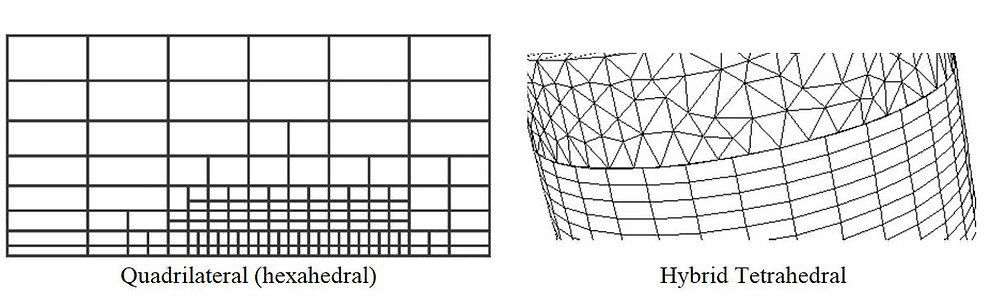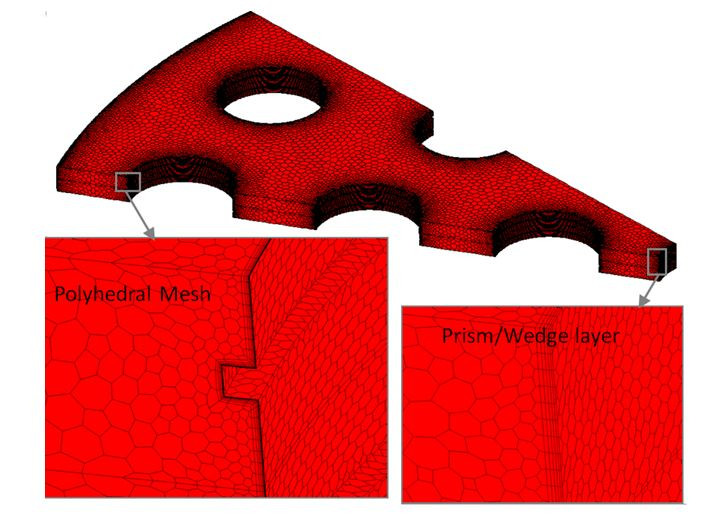Search

# All there is to know about different mesh types in CFD!

1. Grid/Mesh

A mesh divides a geometry into many elements. These are used by the CFD solver to construct control volumes.

Terminology:

• Cell = control volume into which domain is broken up.

• Node = grid point.

• Cell centre = centre of a cell.

• Edge = boundary of a face.

• Face = boundary of a cell.

• Zone = grouping of nodes, faces, cells

• Domain = group of node, face and cell zones.Points to consider when generating a mesh are:

• Mesh resolution

• Type of mesh

• Computer resources

The shapes of control volumes depend on the capabilities of the solver. Structured-grid codes use quadrilaterals in 2D and hexahedrons in 3D flows. Unstructured-grid solvers often use triangles (2D) or tetrahedron (3D), but newer codes can use arbitrary polyhedrons.2. Grid Structure

The purpose of the mesh generator is to decompose the flow domain into control volumes.

The primary outputs are:

• cell vertices

• connectivity information

Precisely where the nodes are relative to the vertices depends on whether the solver uses, for example, cell-centred or cell-vertex storage. Further complexity is introduced if a staggered velocity grid is employed.3. Grid Types

1) Structured Grids:

a) Cartesian

b) Curvilinear

Grids can be Cartesian or curvilinear (usually body-fitting). In the former, grid lines are always parallel to the coordinate axes. In the latter, coordinate surfaces are curved to fit boundaries. There is an alternative division into orthogonal and non-orthogonal grids. In orthogonal grids (for example, Cartesian or polar meshes) all grid lines cross at 90º. Some flows can be treated as axisymmetric, and in these cases, the flow equations can be expressed in terms of polar coordinates (r,θ), rather than Cartesian coordinates (x,y), with minor modifications.2) Block-structured Grids:

a) Matching

b) Non-matching

c) Chimera (composite)

In multi-block structured grids the domain is decomposed into a small number of regions, in each of which the mesh is structured (i.e. cells can be indexed by (i,j,k)). A common arrangement is that grid lines match at the interface between two blocks, so that there are cell vertices that are common to two blocks i.e. matching cells. In some cases, the cell counts do not match at the interface i.e. non-matching cells. Non-matching cells (which could be 2 to 1, 3 to 2, …) should be avoided as much as possible as they tend to increase the computational time. Some solvers also allow overlapping blocks (i.e. chimera grids) where cell vertices do not align. Interpolation is then needed at the boundaries of blocks.Generally, multiple blocks are useful in maintaining a structured grid configuration around complex boundaries. There are no hard and fast rules, but it is generally desirable to avoid sharp changes in grid direction (which lead to lower accuracy) in important and rapidly changing regions of the flow, such as near solid boundaries. One should also strive to minimise the non-orthogonality of the grid.

3) Unstructured Grids:

a) Triangular (tetrahedral)

c) Polygon (polyhedral)

d) Hybrid

Unstructured meshes can accommodate completely arbitrary geometries. However, there are significant penalties to be paid for this flexibility, both in terms of the connectivity data structures and solution algorithms. Grid generators and plotting routines for such meshes are also very complex.

• For flow-aligned problems:

- Quad/hex meshes can provide higher quality solutions with fewer cells/nodes than a comparable tri/tet mesh.

- Quad/hex meshes show reduced numerical diffusion when the mesh is aligned with the flow.

- It does require more effort to generate a quad/hex mesh.

• For complex geometries:

- It would be impractical to generate a structured (flow-aligned) hex mesh.

- You can save meshing effort by using a tri/tet mesh or hybrid mesh which are quicker to generate.

• Flow, however, is generally not aligned with the mesh.

• Hybrid meshes typically combine tri/tet elements with other elements in selected regions

- For example, use wedge/prism elements to resolve boundary layers.

- More efficient and accurate than tri/tet alone.## Recent Posts

See All

1. Modes of Heat Transfer Conduction, convection and radiation are the three mechanisms by which thermal energy may be transferred from one point in space (and time) to another. • Conduction: diffusio